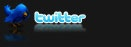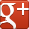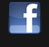top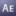# Dynamic Timecode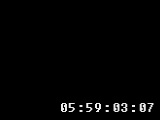Expression to generate a dynamic Timecode on a Text Layer
After Effects CS3
Text-FX
Expressions

Views: 12131
Apply this expression to the source text property of a text layer to get an accurate timecode.

modify the following variables for your needs.
h - hours.
f - frames.
s - seconds.
m - minutes.
``````h=5;m=59;s=3;f=7;
f+=timeToFrames();
s+=Math.floor(f*thisComp.frameDuration);
m+=Math.floor(s/60);
h+=Math.floor(m/60);
f=f%(1/thisComp.frameDuration);
s=s%60;
m=m%60;
if(f<10) {f="0"+f}
if(s<10) {s="0"+s}
if(m<10) {m="0"+m}
if(h<10) {h="0"+h}
h+":"+m+":"+s+":"+f``````
by Oscarus

### Keywords

Timecode

(Min. Version: After Effects CS3, Category: Text-FX, Type: Expressions)
posted by Indy on Mar 08, 2010

## Related topic:# Clockworks Preset/Custom Effect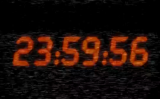With this Preset, you can create Clocks, or countdowns. You can add Time, with fully functional switches and parameters!
After Effects CS3
Text-FX
Presets (ffx)

Views: 13020
posted by MaxX on Feb 02, 2010